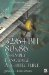Function yMAX(p, q) MaximumFunction y=MAX(p, q) 'Maximum'

The function MAX() would have a similar implementation except that p and q would be internally swapped as shown below.

MAX PROC C PUBLIC p:DWORD, q:DWORD     mov edx,q               ; edx = q     mov ecx,p               ; ecx = p             ; r=(q < p) ? q : p         ; r = (qp) >> INT_MAX_BITS;         ; ()=0xFFFFFFFF (+)=0x00000000     mov eax,edx     sub eax,ecx     sar eax,INT_MAX_BITS    ; (int >> 31)             ; return (q   r)   (p   (32/64-Bit 80x86 Assembly Language Architecture
ISBN: 1598220020
EAN: 2147483647
Year: 2003
Pages: 191

Similar book on Amazon